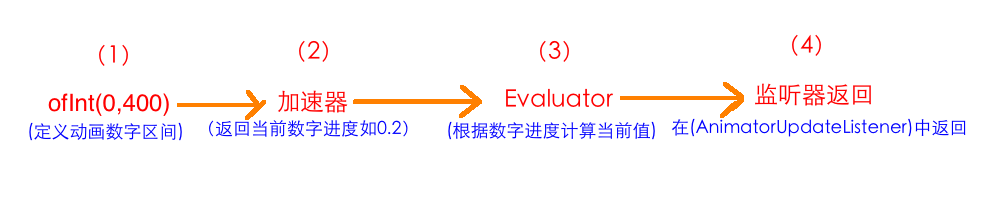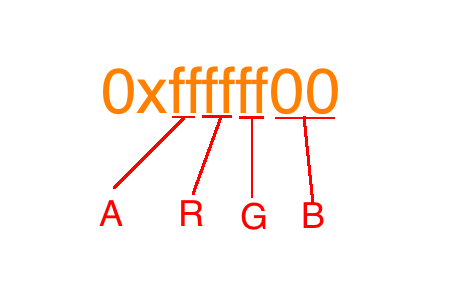# Android自定义控件：动画类（六）----ValueAnimator高级进阶（一）

## 一、插值器

### 1、使用插值器[java] view plain
1. ValueAnimator animator = ValueAnimator.ofInt(0,600);
2.
3. animator.addUpdateListener(new ValueAnimator.AnimatorUpdateListener() {
4.     @Override
5.     public void onAnimationUpdate(ValueAnimator animation) {
6.         int curValue = (int)animation.getAnimatedValue();
7.         tv.layout(tv.getLeft(),curValue,tv.getRight(),curValue+tv.getHeight());
8.     }
9. });
10. animator.setDuration(1000);
11. animator.setInterpolator(new BounceInterpolator());
12. animator.start();

### 2、自定义加速器

#### 1、概述

[java] view plain
1. public class LinearInterpolator implements Interpolator {
2.
3.     public LinearInterpolator() {
4.     }
5.
6.     public LinearInterpolator(Context context, AttributeSet attrs) {
7.     }
8.
9.     public float getInterpolation(float input) {
10.         return input;
11.     }
12. }
13. public interface Interpolator extends TimeInterpolator {
14. }
LinearInterpolator实现了Interpolator接口；而Interpolator接口则直接继承自TimeInterpolator，而且并没有添加任何其它的方法。

[java] view plain
1. /**
2.  * A time interpolator defines the rate of change of an animation. This allows animations
3.  * to have non-linear motion, such as acceleration and deceleration.
4.  */
5. public interface TimeInterpolator {
6.
7.     /**
8.      * Maps a value representing the elapsed fraction of an animation to a value that represents
9.      * the interpolated fraction. This interpolated value is then multiplied by the change in
10.      * value of an animation to derive the animated value at the current elapsed animation time.
11.      *
12.      * @param input A value between 0 and 1.0 indicating our current point
13.      *        in the animation where 0 represents the start and 1.0 represents
14.      *        the end
15.      * @return The interpolation value. This value can be more than 1.0 for
16.      *         interpolators which overshoot their targets, or less than 0 for
17.      *         interpolators that undershoot their targets.
18.      */
19.     float getInterpolation(float input);
20. }

[java] view plain
1. ValueAnimator anim = ValueAnimator.ofInt(100400);
2. anim.setDuration(1000);
3. anim.addUpdateListener(new ValueAnimator.AnimatorUpdateListener() {
4.     @Override
5.     public void onAnimationUpdate(ValueAnimator animation) {
6.         float currentValue = (float) animation.getAnimatedValue();
7.         Log.d("TAG""cuurent value is " + currentValue);
8.     }
9. });
10. anim.start();

[java] view plain
1. public class LinearInterpolator implements Interpolator {
2.
3.     …………
4.
5.     public float getInterpolation(float input) {
6.         return input;
7.     }
8. }

#### 2、示例

[java] view plain
1. public class MyInterploator implements TimeInterpolator {
2.     @Override
3.     public float getInterpolation(float input) {
4.         return 1-input;
5.     }
6. }

[java] view plain
1. ValueAnimator animator = ValueAnimator.ofInt(0,600);
2.
3. animator.addUpdateListener(new ValueAnimator.AnimatorUpdateListener() {
4.     @Override
5.     public void onAnimationUpdate(ValueAnimator animation) {
6.         int curValue = (int)animation.getAnimatedValue();
7.         tv.layout(tv.getLeft(),curValue,tv.getRight(),curValue+tv.getHeight());
8.     }
9. });
10. animator.setDuration(1000);
11. animator.setInterpolator(new MyInterploator());
12. animator.start();## 二、Evaluator

### 1、概述(1)、ofInt(0,400)表示指定动画的数字区间，是从0运动到400；
(2)、加速器：上面我们讲了，在动画开始后，通过加速器会返回当前动画进度所对应的数字进度，但这个数字进度是百分制的，以小数表示，如0.2
(3)、Evaluator:我们知道我们通过监听器拿到的是当前动画所对应的具体数值，而不是百分制的进度。那么就必须有一个地方会根据当前的数字进度，将其转化为对应的数值，这个地方就是Evaluator；Evaluator就是将从加速器返回的数字进度转成对应的数字值。所以上部分中，我们讲到的公式：

[java] view plain
1. 当前的值 = 100 + （400 - 100）* 显示进度

（4）、监听器：我们通过在AnimatorUpdateListener监听器使用animation.getAnimatedValue()函数拿到Evaluator中返回的数字值。

### 2、各种Evaluator

[java] view plain
1. ValueAnimator animator = ValueAnimator.ofInt(0,600);
2.
3. animator.addUpdateListener(new ValueAnimator.AnimatorUpdateListener() {
4.     @Override
5.     public void onAnimationUpdate(ValueAnimator animation) {
6.         int curValue = (int)animation.getAnimatedValue();
7.         tv.layout(tv.getLeft(),curValue,tv.getRight(),curValue+tv.getHeight());
8.     }
9. });
10. animator.setDuration(1000);
11. animator.setEvaluator(new IntEvaluator());
12. animator.setInterpolator(new BounceInterpolator());
13. animator.start();

[java] view plain
1. /**
2.  * This evaluator can be used to perform type interpolation between <code>int</code> values.
3.  */
4. public class IntEvaluator implements TypeEvaluator<Integer> {
5.
6.     /**
7.      * This function returns the result of linearly interpolating the start and end values, with
8.      * <code>fraction</code> representing the proportion between the start and end values. The
9.      * calculation is a simple parametric calculation: <code>result = x0 + t * (v1 - v0)</code>,
10.      * where <code>x0</code> is <code>startValue</code>, <code>x1</code> is <code>endValue</code>,
11.      * and <code>t</code> is <code>fraction</code>.
12.      *
13.      * @param fraction   The fraction from the starting to the ending values
14.      * @param startValue The start value; should be of type <code>int</code> or
15.      *                   <code>Integer</code>
16.      * @param endValue   The end value; should be of type <code>int</code> or <code>Integer</code>
17.      * @return A linear interpolation between the start and end values, given the
18.      *         <code>fraction</code> parameter.
19.      */
20.     public Integer evaluate(float fraction, Integer startValue, Integer endValue) {
21.         int startInt = startValue;
22.         return (int)(startInt + fraction * (endValue - startInt));
23.     }
24. }

startValue和endValue分别对应ofInt(int start,int end)中的start和end的数值；

[java] view plain
1. return (int)(startInt + fraction * (endValue - startInt));

[java] view plain
1. 当前的值 = 100 + （400 - 100）* 显示进度

### 3、自定义Evalutor

#### （1）、简单实现MyEvalutor

[java] view plain
1. public class MyEvaluator implements TypeEvaluator<Integer> {
2.     @Override
3.     public Integer evaluate(float fraction, Integer startValue, Integer endValue) {
4.         return null;
5.     }
6. }

[java] view plain
1. public class MyEvaluator implements TypeEvaluator<Integer> {
2.     @Override
3.     public Integer evaluate(float fraction, Integer startValue, Integer endValue) {
4.         int startInt = startValue;
5.         return (int)(200+startInt + fraction * (endValue - startInt));
6.     }
7. }

[java] view plain
1. ValueAnimator animator = ValueAnimator.ofInt(0,400);
2.
3. animator.addUpdateListener(new ValueAnimator.AnimatorUpdateListener() {
4.     @Override
5.     public void onAnimationUpdate(ValueAnimator animation) {
6.         int curValue = (int)animation.getAnimatedValue();
7.         tv.layout(tv.getLeft(),curValue,tv.getRight(),curValue+tv.getHeight());
8.     }
9. });
10. animator.setDuration(1000);
11. animator.setEvaluator(new MyEvaluator());
12. animator.start();#### （2）、实现倒序输出实例

[java] view plain
1. public class ReverseEvaluator implements TypeEvaluator<Integer> {
2.     @Override
3.     public Integer evaluate(float fraction, Integer startValue, Integer endValue) {
4.         int startInt = startValue;
5.         return (int) (endValue - fraction * (endValue - startInt));
6.     }
7. }

[java] view plain
1. ValueAnimator animator = ValueAnimator.ofInt(0,400);
2.
3. animator.addUpdateListener(new ValueAnimator.AnimatorUpdateListener() {
4.     @Override
5.     public void onAnimationUpdate(ValueAnimator animation) {
6.         int curValue = (int)animation.getAnimatedValue();
7.         tv.layout(tv.getLeft(),curValue,tv.getRight(),curValue+tv.getHeight());
8.     }
9. });
10. animator.setDuration(1000);
11. animator.setEvaluator(new ReverseEvaluator());
12. animator.start();### 4、关于ArgbEvalutor

#### 1、使用ArgbEvalutor

ArgbEvalutor是用来做颜色值过渡转换的。可能是谷歌的开发人员觉得大家对颜色值变换可能并不知道要怎么做，所以特地给我们提供了这么一个过渡Evalutor；

[java] view plain
1. public class ArgbEvaluator implements TypeEvaluator {
2.     public Object evaluate(float fraction, Object startValue, Object endValue) {
3.         int startInt = (Integer) startValue;
4.         int startA = (startInt >> 24);
5.         int startR = (startInt >> 16) & 0xff;
6.         int startG = (startInt >> 8) & 0xff;
7.         int startB = startInt & 0xff;
8.
9.         int endInt = (Integer) endValue;
10.         int endA = (endInt >> 24);
11.         int endR = (endInt >> 16) & 0xff;
12.         int endG = (endInt >> 8) & 0xff;
13.         int endB = endInt & 0xff;
14.
15.         return (int)((startA + (int)(fraction * (endA - startA))) << 24) |
16.                 (int)((startR + (int)(fraction * (endR - startR))) << 16) |
17.                 (int)((startG + (int)(fraction * (endG - startG))) << 8) |
18.                 (int)((startB + (int)(fraction * (endB - startB))));
19.     }
20. }

[java] view plain
1. ValueAnimator animator = ValueAnimator.ofInt(0xffffff00,0xff0000ff);
2. animator.setEvaluator(new ArgbEvaluator());
3. animator.setDuration(3000);
4.
5. animator.addUpdateListener(new ValueAnimator.AnimatorUpdateListener() {
6.     @Override
7.     public void onAnimationUpdate(ValueAnimator animation) {
8.         int curValue = (int)animation.getAnimatedValue();
9.         tv.setBackgroundColor(curValue);
10.
11.     }
12. });
13.
14. animator.start();#### 2、ArgbEvalutor的实现原理

[java] view plain
1. /**
2.  * This evaluator can be used to perform type interpolation between integer
3.  * values that represent ARGB colors.
4.  */
5. public class ArgbEvaluator implements TypeEvaluator {
6.
7.     /**
8.      * This function returns the calculated in-between value for a color
9.      * given integers that represent the start and end values in the four
10.      * bytes of the 32-bit int. Each channel is separately linearly interpolated
11.      * and the resulting calculated values are recombined into the return value.
12.      *
13.      * @param fraction The fraction from the starting to the ending values
14.      * @param startValue A 32-bit int value representing colors in the
15.      * separate bytes of the parameter
16.      * @param endValue A 32-bit int value representing colors in the
17.      * separate bytes of the parameter
18.      * @return A value that is calculated to be the linearly interpolated
19.      * result, derived by separating the start and end values into separate
20.      * color channels and interpolating each one separately, recombining the
21.      * resulting values in the same way.
22.      */
23.     public Object evaluate(float fraction, Object startValue, Object endValue) {
24.         int startInt = (Integer) startValue;
25.         int startA = (startInt >> 24);
26.         int startR = (startInt >> 16) & 0xff;
27.         int startG = (startInt >> 8) & 0xff;
28.         int startB = startInt & 0xff;
29.
30.         int endInt = (Integer) endValue;
31.         int endA = (endInt >> 24);
32.         int endR = (endInt >> 16) & 0xff;
33.         int endG = (endInt >> 8) & 0xff;
34.         int endB = endInt & 0xff;
35.
36.         return (int)((startA + (int)(fraction * (endA - startA))) << 24) |
37.                 (int)((startR + (int)(fraction * (endR - startR))) << 16) |
38.                 (int)((startG + (int)(fraction * (endG - startG))) << 8) |
39.                 (int)((startB + (int)(fraction * (endB - startB))));
40.     }
41. }

[java] view plain
1. int startInt = (Integer) startValue;
2. int startA = (startInt >> 24);
3. int startR = (startInt >> 16) & 0xff;
4. int startG = (startInt >> 8) & 0xff;
5. int startB = startInt & 0xff;[java] view plain
1. int endInt = (Integer) endValue;
2. int endA = (endInt >> 24);
3. int endR = (endInt >> 16) & 0xff;
4. int endG = (endInt >> 8) & 0xff;
5. int endB = endInt & 0xff;

[java] view plain
1. startA + (int)(fraction * (endA - startA)))

startR + (int)(fraction * (endR - startR)表示当前进度下的红色值
startG + (int)(fraction * (endG - startG))表示当前进度下的绿色值
startB + (int)(fraction * (endB - startB))表示当前进度下的蓝色值

posted @ 2016-08-31 11:48  vegatate  阅读(251)  评论(0编辑  收藏  举报